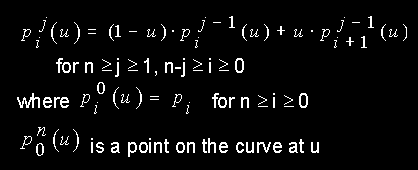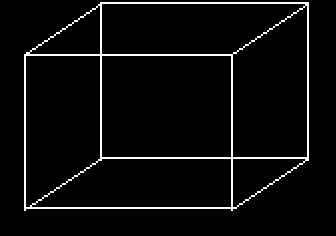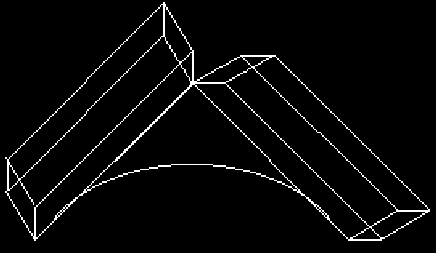# 3D Free-form Deformation

by Suzanne Martin

Free-form deformation (FFD) is an important tool in computer-assisted geometric design and animation. Barr [BARR84] developed a method which altered the transformation while it was being applied to the object. A more general technique was later developed by Sederberg and Parry [SEDE86] which deformed objects by deforming the space in which the object is embedded. Another method was developed by Coquillart [COQU90] which used an initial lattice and B-spline control points to approximate the shape of the intended deformation. Below is a brief discussion of the above methods followed by an explanation of a technique developed by Chang and Rockwood [CHAN94].

## Preliminary

### Barr Technique

A deformation technique developed by Alan Barr [BARR84] uses a set of hierarchical transformations for deforming an object. This includes stretching, bending, twisting, and taper operators. This technique uses the surface normal vector of the undeformed surface and a transformation matrix to calculate the normal vector of an arbitrarily deformed smooth surface. Complex objects can be created from simpler ones since the deformations are easily combined in a hierarchical structure. The position vector and normal vector in the more complex objects are calculated from the position vector and normal vector in simpler objects. Each level in the deformation hierarchy requires an additional matrix multiply for the normal vector calculation.

### Sederberg and Parry Technique

Sederberg and Parry's technique [SEDE86] deforms solid geometric modes in a free-form manner. Surface primitives of any type or degree can be deformed; for example: planes, quadrics, parametric surface patches, or implicitly defined surfaces. This technique is based on trivariate Bernstein polynomials. It enables deformation of objects by manipulating the control points. This free-form deformation technique can be thought of as a method for sculpturing models.

### Coquillart Technique

Coquillart [COQU90] developed the Extended Free-Form Deformation, EFFD, as an extension of Sederberg and Parry's technique. The goal of this technique is to change the shape of an existing surface either by bending it along an arbitrarily shaped curve or by adding randomly shaped bumps to it. It uses non-parallelepiped type 3D lattices. The user defines the shape of the lattice which in turn determines the shape of the deformation.

## Chang and Rockwood Approach

This approach developed by Chang and Rockwood [CHAN94] deforms an object by repeatedly applying affine transformations in space. The user defines the curve that the object warps along. It uses a single Bézier curve and a few affine maps controlled by "handles". It generalizes the de Casteljau algorithm for curve evaluation.

The de Casteljau Algorithm for evaluating a Bézier curve of degree n with control pointsat parameter u is:.

The Chang and Rockwood method takes the above repeated univarite linear interpolation and generalizes it to a trivariate method. A local coordinate system is defined for each segment of the Bézier control polygon. It is defined at one endpoint by 2 user-specified axes, called "handles". The objects are then mapped along an embedded Bézier curve by applying iterative affine transformations using the handles and control polygon segments. The following four figures are an example of this:

```Figure 1: Quadratic Bézier curve, control polygon and
two user-specified axes

```

```Figure 2: Cube to be deformed

```

```Figure 3: First-level execution of the generalized
de Casteljau algorithm the cube is mapped
affinely to each segment of the control
polygon

```

```Figure 4: Second-level execution of the generalized de Casteljau
algorithm the original cube is warped along the Bézier curve

```

### Deformation Algorithm

In 3-space each iteration of the de Casteljau algorithm is an execution of a degenerate affine transformation. It maps space to a line.

Let vectors r, s, t span affine space. Function Ø[p,q]: R^3 -> R^3 is then defined as an affine transformation from parameter space into homogeneous form

```       |u|   |qx - px  sx  tx  px|  |u|     |x|
Ø[p,q] |v| = |qy - py  sy  ty  py|  |v|  =  |y|
|w|   |qz - pz  sz  tz  pz|  |w|     |z|
|l|   |   0     0   0   1 |  |1|     |1|

where r_vector = (qx - px, qy - py, qz - pz), s_vector = (sx, sy, sz) and
t_vector = (tx, ty, tz).

```

The generalized de Casteljau algorithm is:

```for 0 <= i <= n,= Ø [,] (u) for 1 <= j <= n, 0 <= i <= n-j,

where n is the degree andis the deformed point on the
object at u_vector = (u, v, w).  Iterative affine transformations
are applied resulting in the Bézier curve being part of the
deformation space.

The result of an iterative affine transformation:

Level 1 of the generalized de Casteljau algorithm:

If the s_vector in the transformation matrix equals a
0 vector, then the solid will be mapped into just a surface
patch.  The depth information is lost.

If the t_vector equals a 0 vector then the degeneration
is similar to the s_vector as described above, but
degeneration occurs on a different axis.

If both the s_vector and the t_vector equal 0 vectors, then
the deformation process becomes the classic de Casteljau
algorithm.  Since parameters v and w are no longer
effective, the solid is mapped to the curve.

Levels 2 and higher:

If the s_vector and the t_vector are all zero, the function
blends the result of the first-level execution linearly.

If sx, sy or sz is nonzero, the deformed object is sheared
by an amount proportional to the v value along the direction
of (sx,0,0) or (0,sy,0) or (0,0,sz).

The same applies to the t_vector, but shearing proportional
to w instead of v.  The effects of shearing are hierarchical.
For example: there are 3 levels of affine transformations in
the cubic case.  A nonzero s_vector or t_vector in the
second-level transformation matrix, shears only the portion
of the object related to the appropriate control polygon
segments.  A nonzero s_vector or t_vector in the third-level
affects the whole object.

```

## Conclusion

This approach is a simple design scheme for an extensively useful set of deformations. It is a more efficient algorithm because it uses affine transformation and a univariate interpolation in space as opposed to an algorithm that iterates over three variables.

Below is a comparison of the computation times between these methods.

```-------------------------------------------------------------------------------------
|                    |                    |
Deformation\ Degree   |       Linear       |     Quadratic      |       Cubic
|                    |                    |
=====================================================================================
|                    |                    |
Bézier lattice method | 42 multiplications | 90 multiplications | 144 multiplications
|                    |                    |
Sederberg and Parry   |                    |                    |
|                    |                    |
----------------------|--------------------|--------------------|--------------------
|                    |                    |
Generalized de        |                    |                    |
Casteljau method      |  9 multiplications | 27 multiplications | 54 multiplications
|                    |                    |
|                    |                    |
----------------------|--------------------|--------------------|--------------------
```

## References

[BARR84] Barr, A. H., Global and Local Deformations of Solid Primitives, Proceedings of SIGGRAPH ‘84, Computer Graphics 18, 3 (July 1984), 21-30.

[CHAN94] Chang, Y.and Rockwood, A. P., A Generalized de Casteljau Approach to 3D Free-form Deformation, Proceedings of SIGGRAPH ‘94, Computer Graphics (July 1994), 257-260.

[COQU90] Coquillart, S., Extended Free-Form Deformation: A sculpturing Tool for 3D Geometric Modeling, Proceedings of SIGGRAPH ‘90, Computer Graphics 24, 4 (August 1990), 187-196.

[SEDE86] Sederberg, T. W. and Parry, S. R., Free-Form Deformation of Solid Geometric Models, Proceedings of SIGGRAPH ‘86, Computer Graphics 20, 4 (August 1986), 151-159.

matt@owl.WPI.EDU